# Chapter 5 Analog Transmission Kyung Hee University 1

• Slides: 57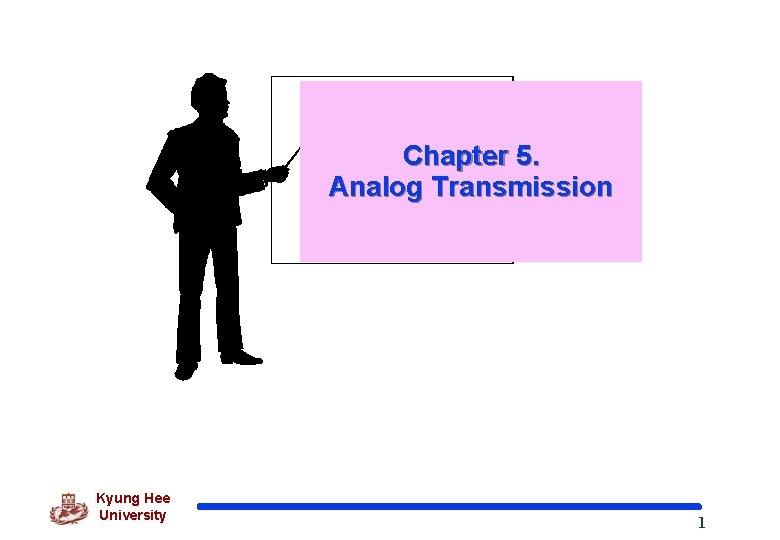Chapter 5. Analog Transmission Kyung Hee University 15. 1 Digital-to-Analog 부호화 q ASK(Amplitude Shift Keying) q FSK(Frequency Shift Keying) q PSK(Phase Shift Keying) Shift Keying = modulation q QAM(Quadrature Amplitude Modulation) : related to Amplitude and Phase Kyung Hee University 2Digital-to-Analog 부호화(cont’d) q Type of Digital-to-Analog encoding Kyung Hee University 3Digital-to-Analog 부호화(cont’d) q Bit rate : the number of bits per second. q Baud rate : the number of signal units per second. Baud rate is less than or equal to the bit rate. Bit rate equals the baud rate x the number of bits represented by each signal unit q 반송신호 또는 주파수 (Carrier Signal or Carrier Frequency) base signal for the information signal Kyung Hee University 4Digital-to-Analog 부호화(cont’d) q Example 1 An analog signal carries 4 bits in each signal element. If 1000 signal elements are sent per second, find the baud rate and the bit rate. q Solution Baud rate = Number of signal elements = 1000 bauds per second Bit rate = Baud rate x Number of bits per signal element = 1000 x 4 = 4000 bps Kyung Hee University 5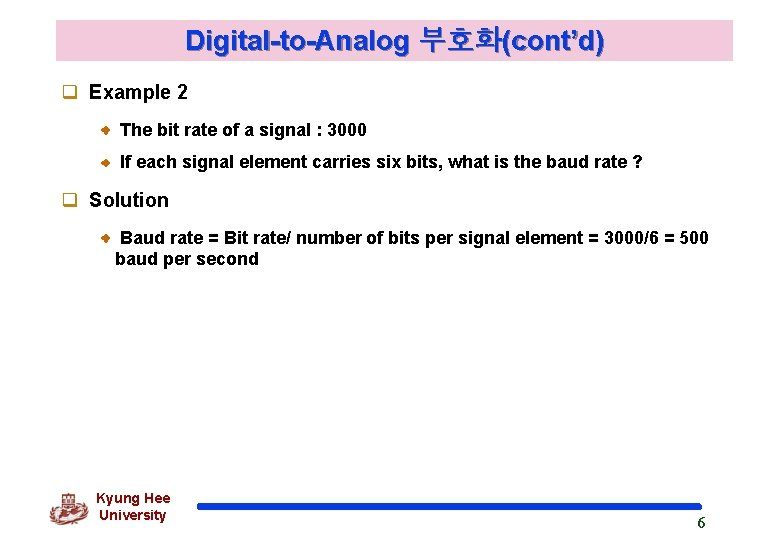Digital-to-Analog 부호화(cont’d) q Example 2 The bit rate of a signal : 3000 If each signal element carries six bits, what is the baud rate ? q Solution Baud rate = Bit rate/ number of bits per signal element = 3000/6 = 500 baud per second Kyung Hee University 6Digital-to-Analog 부호화(cont’d) q. ASK(Amplitude Shift Keying) Both frequency and phase remain constant while the amplitude changes. Highly susceptible to noise interference l Noise usually affects the amplitude. Kyung Hee University 7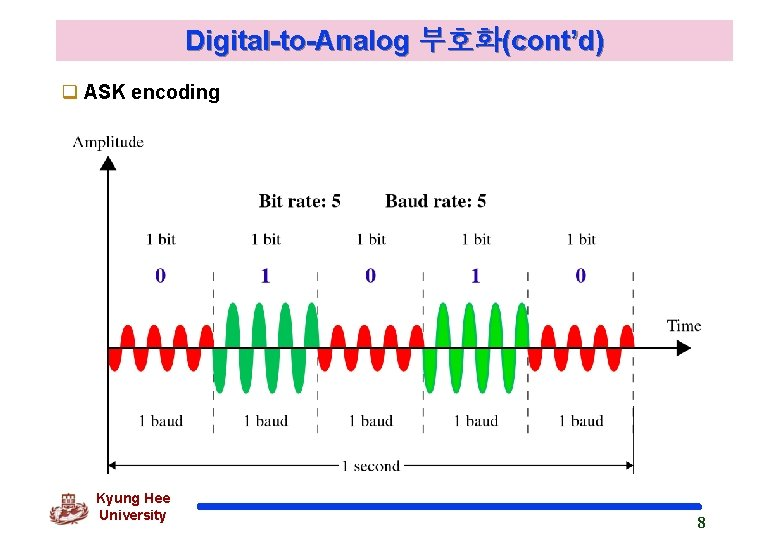Digital-to-Analog 부호화(cont’d) q ASK encoding Kyung Hee University 8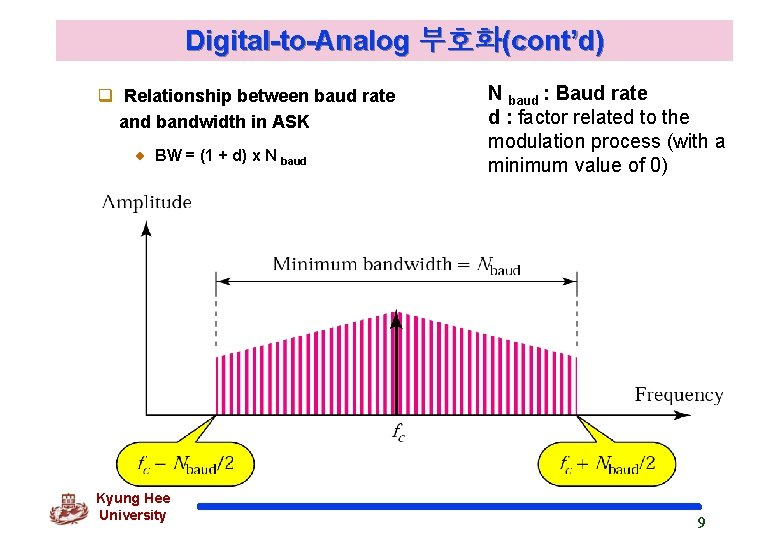Digital-to-Analog 부호화(cont’d) q Relationship between baud rate and bandwidth in ASK BW = (1 + d) x N baud Kyung Hee University N baud : Baud rate d : factor related to the modulation process (with a minimum value of 0) 9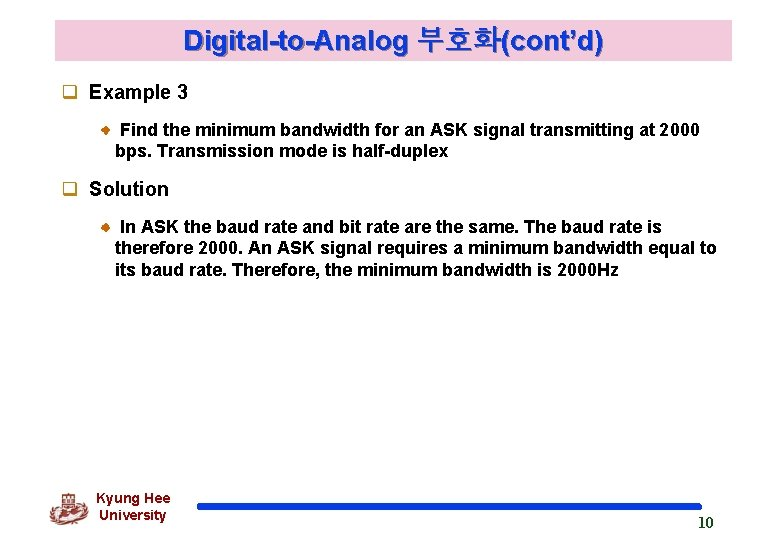Digital-to-Analog 부호화(cont’d) q Example 3 Find the minimum bandwidth for an ASK signal transmitting at 2000 bps. Transmission mode is half-duplex q Solution In ASK the baud rate and bit rate are the same. The baud rate is therefore 2000. An ASK signal requires a minimum bandwidth equal to its baud rate. Therefore, the minimum bandwidth is 2000 Hz Kyung Hee University 10Digital-to-Analog 부호화(cont’d) q Example 4 Given a bandwidth of 5000 Hz for an ASK signal, what are the baud rate and bit rate? q Solution In ASK the baud rate is the same as the bandwidth, which means the baud rate is 5000. But because the baud rate and the bit rate are also the same for ASK, the bit rate is 5000 bps. Kyung Hee University 11Digital-to-Analog 부호화(cont’d) q Example 5 Given a bandwidth of 10, 000 Hz (1000 to 11, 000 Hz), draw the fullduplex ASK diagram of the system. Find the carriers and the bandwidth in each direction. Assume there is no gap between the bands in two directions. q Solution Bandwidth for each direction : 10000/2 = 5000 Hz Carrier frequencies : fc (forward) = 1000 + 5000/2 = 3500 Hz fc (backward) = 11000 – 5000/2 = 8500 Hz Kyung Hee University 12Digital-to-Analog 부호화(cont’d) Solution to Example 5 Kyung Hee University 13Digital-to-Analog 부호화(cont’d) q. FSK(Frequency Shift Keying) The frequency of the signal is varied to represent binary 1 or 0. Avoiding most of the problems from noise - Can ignore voltage spikes Kyung Hee University 14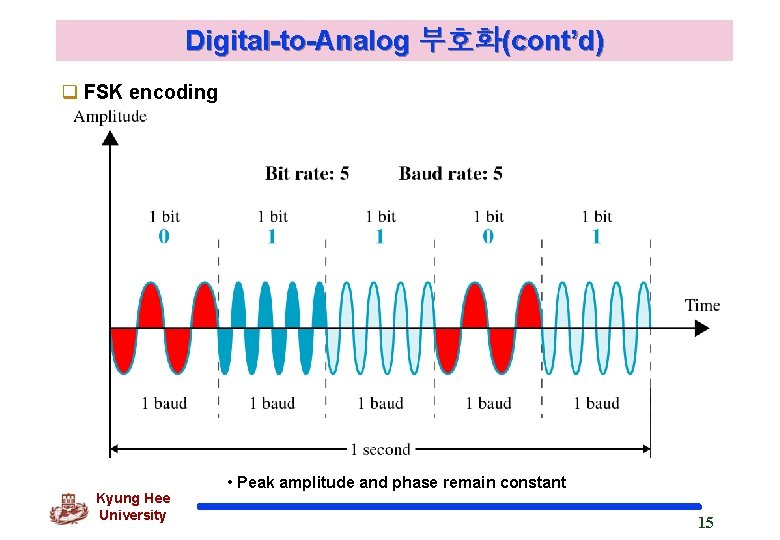Digital-to-Analog 부호화(cont’d) q FSK encoding Kyung Hee University • Peak amplitude and phase remain constant 15Digital-to-Analog 부호화(cont’d) q Bandwidth for FSK Kyung Hee University 16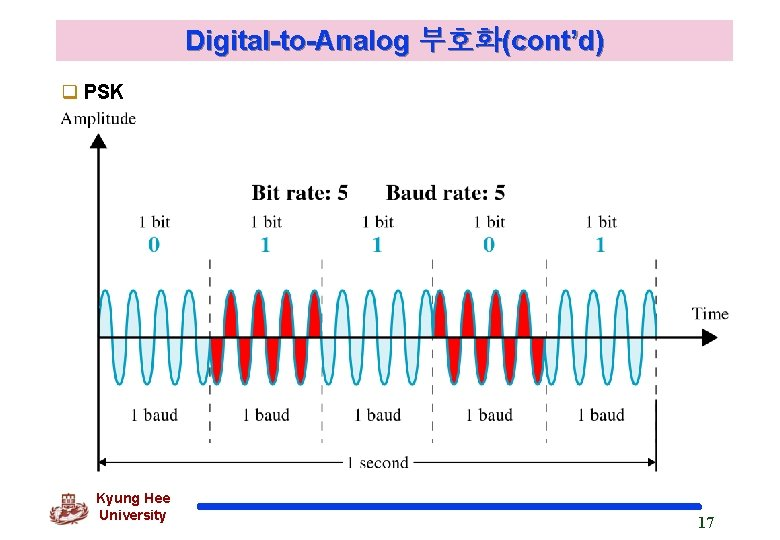Digital-to-Analog 부호화(cont’d) q PSK Kyung Hee University 17Digital-to-Analog 부호화(cont’d) q PSK(Phase Shift Keying) the phase is varied to represent binary 1 or 0. bit phase 0 180 1 0 Constellation diagram Kyung Hee University 18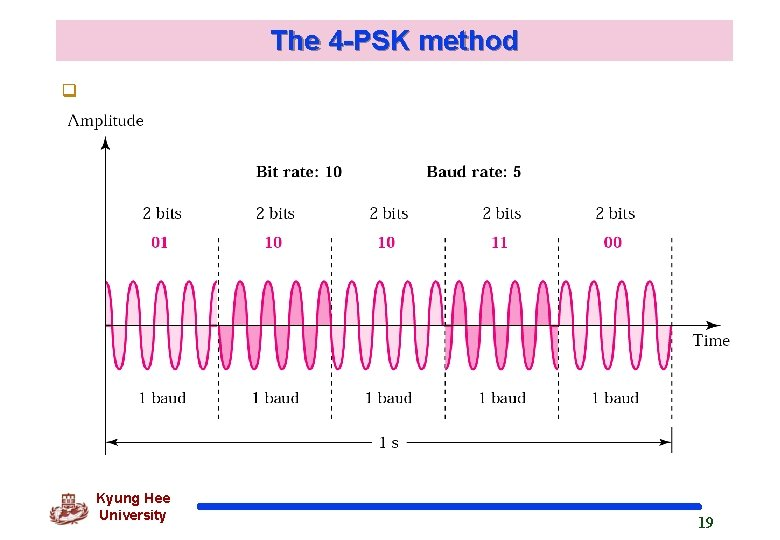The 4 -PSK method q Kyung Hee University 19The 4 -PSK Characteristics Kyung Hee University 20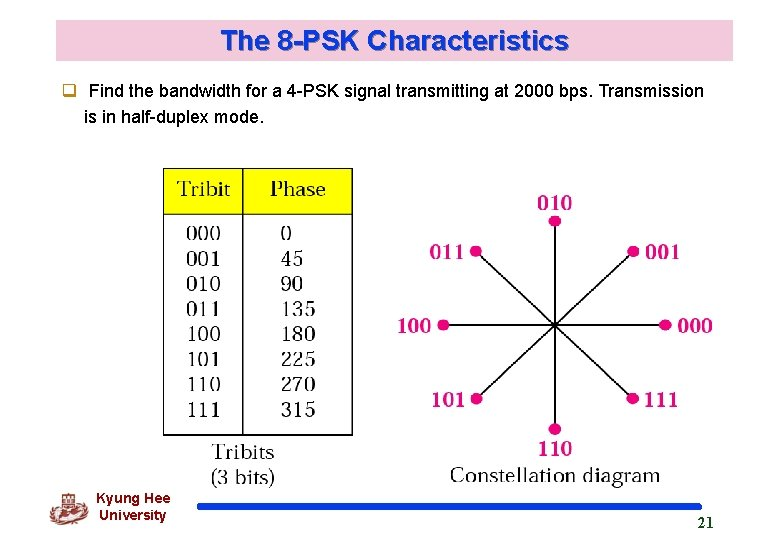The 8 -PSK Characteristics q Find the bandwidth for a 4 -PSK signal transmitting at 2000 bps. Transmission is in half-duplex mode. Kyung Hee University 21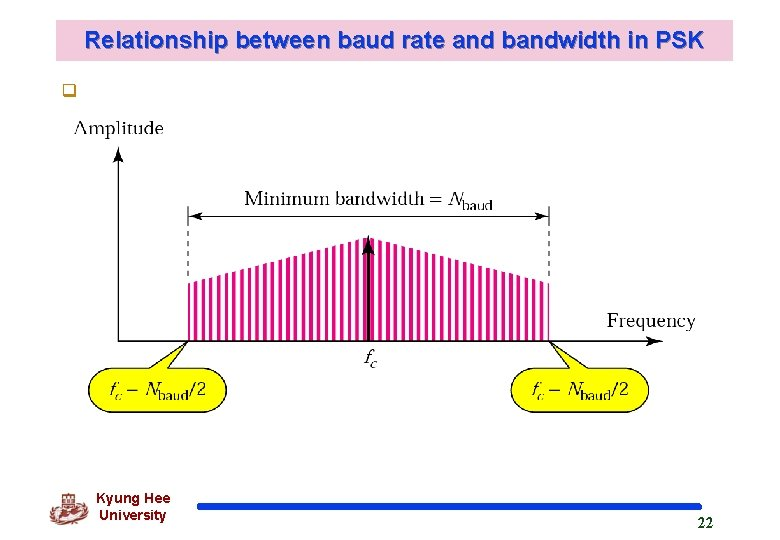Relationship between baud rate and bandwidth in PSK q Kyung Hee University 22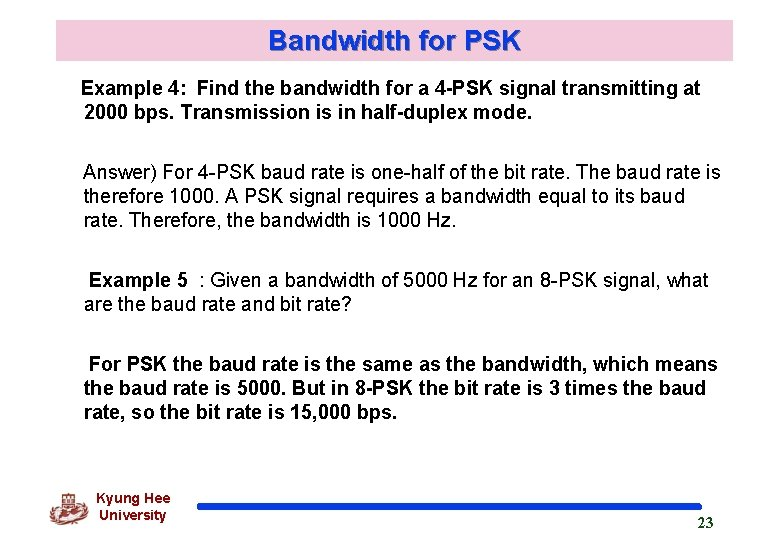Bandwidth for PSK Example 4: Find the bandwidth for a 4 -PSK signal transmitting at 2000 bps. Transmission is in half-duplex mode. Answer) For 4 -PSK baud rate is one-half of the bit rate. The baud rate is therefore 1000. A PSK signal requires a bandwidth equal to its baud rate. Therefore, the bandwidth is 1000 Hz. Example 5 : Given a bandwidth of 5000 Hz for an 8 -PSK signal, what are the baud rate and bit rate? For PSK the baud rate is the same as the bandwidth, which means the baud rate is 5000. But in 8 -PSK the bit rate is 3 times the baud rate, so the bit rate is 15, 000 bps. Kyung Hee University 23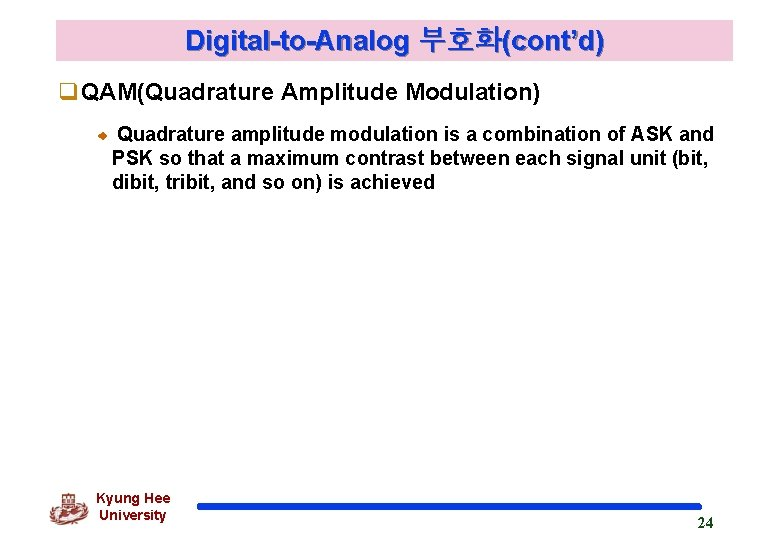Digital-to-Analog 부호화(cont’d) q. QAM(Quadrature Amplitude Modulation) Quadrature amplitude modulation is a combination of ASK and PSK so that a maximum contrast between each signal unit (bit, dibit, tribit, and so on) is achieved Kyung Hee University 24The 4 -QAM and 8 -QAM constellations Kyung Hee University 25Digital-to-Analog 부호화(cont’d) q Time domain for an 8 -QAM signal Kyung Hee University 26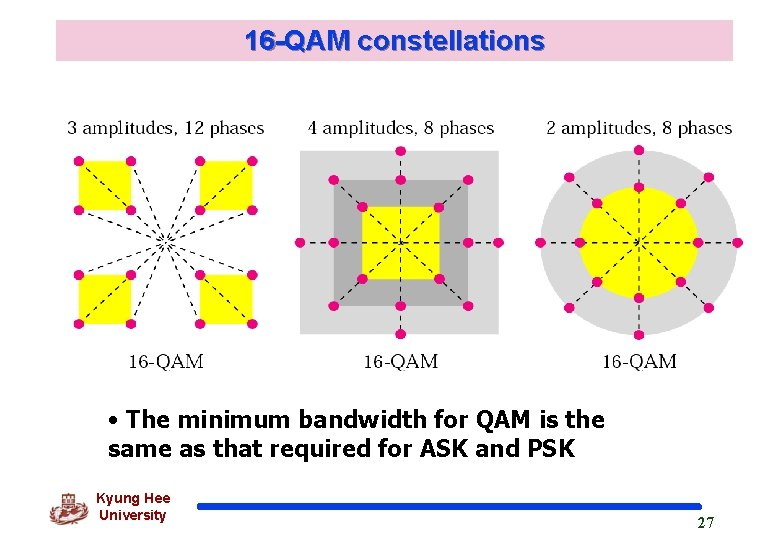16 -QAM constellations • The minimum bandwidth for QAM is the same as that required for ASK and PSK Kyung Hee University 27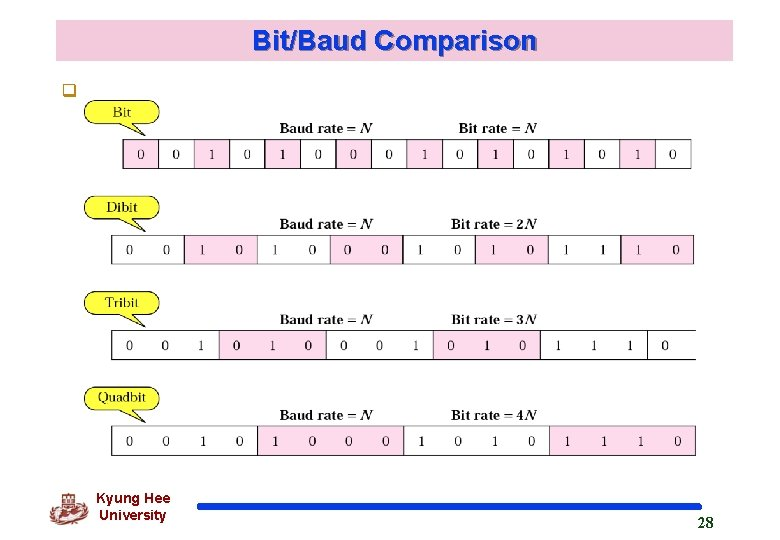Bit/Baud Comparison q Kyung Hee University 28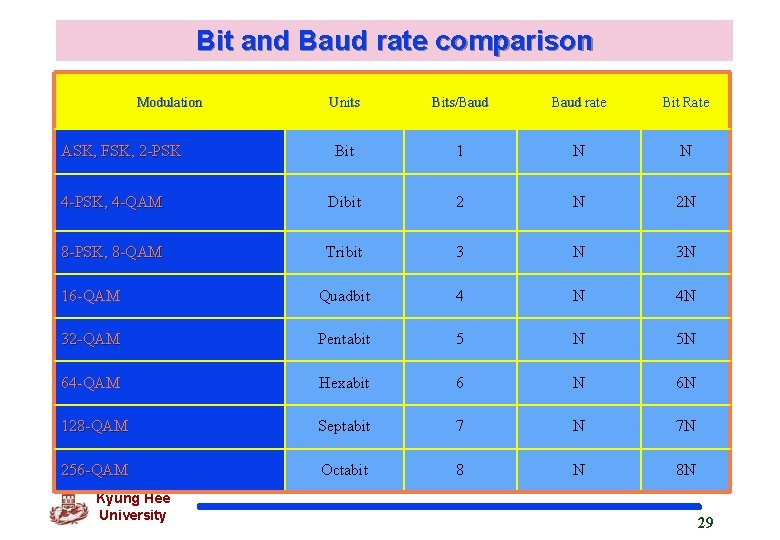Bit and Baud rate comparison Modulation Units Bits/Baud rate Bit Rate Bit 1 N N 4 -PSK, 4 -QAM Dibit 2 N 2 N 8 -PSK, 8 -QAM Tribit 3 N 3 N 16 -QAM Quadbit 4 N 4 N 32 -QAM Pentabit 5 N 5 N 64 -QAM Hexabit 6 N 6 N 128 -QAM Septabit 7 N 7 N 256 -QAM Octabit 8 N 8 N ASK, FSK, 2 -PSK Kyung Hee University 29Bit and Baud rate comparison q Example 10 : A constellation diagram consists of eight equally spaced points on a circle. If the bit rate is 4800 bps, what is the baud rate? q. Solution : The constellation indicates 8 -PSK with the points 45 degrees apart. Since 23 = 8, 3 bits are transmitted with each signal unit. Therefore, the baud rate is 4800 / 3 = 1600 baud Kyung Hee University 30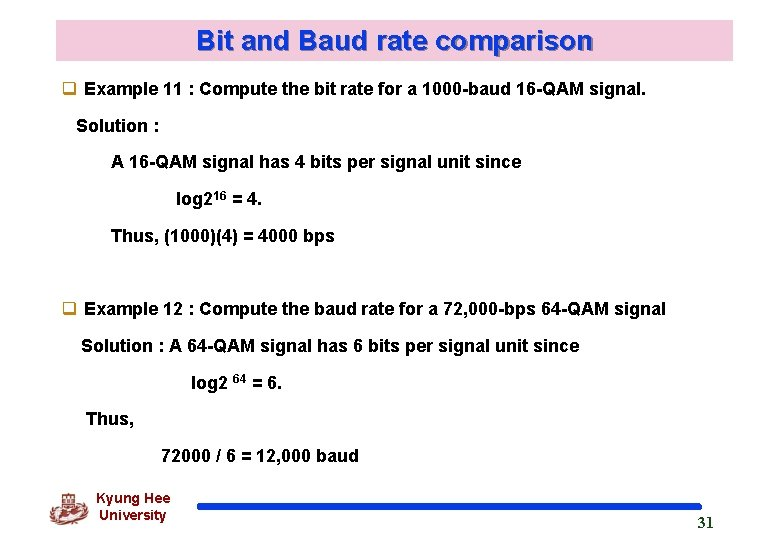Bit and Baud rate comparison q Example 11 : Compute the bit rate for a 1000 -baud 16 -QAM signal. Solution : A 16 -QAM signal has 4 bits per signal unit since log 216 = 4. Thus, (1000)(4) = 4000 bps q Example 12 : Compute the baud rate for a 72, 000 -bps 64 -QAM signal Solution : A 64 -QAM signal has 6 bits per signal unit since log 2 64 = 6. Thus, 72000 / 6 = 12, 000 baud Kyung Hee University 31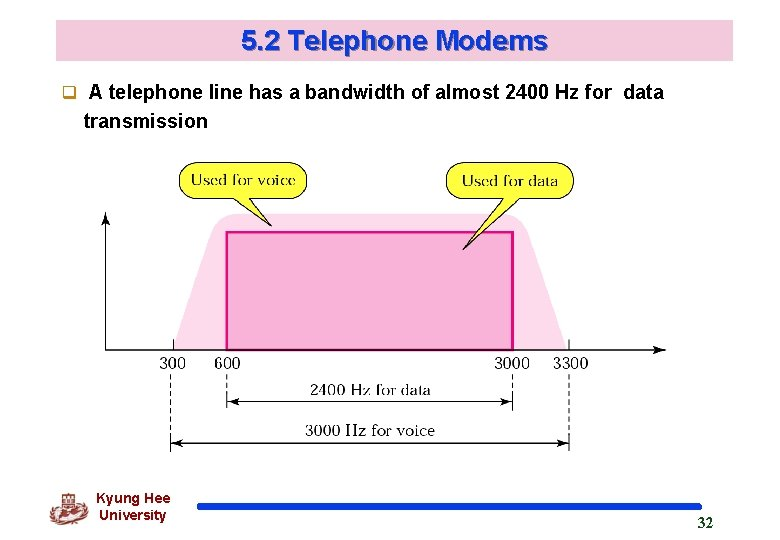5. 2 Telephone Modems q A telephone line has a bandwidth of almost 2400 Hz for data transmission Kyung Hee University 32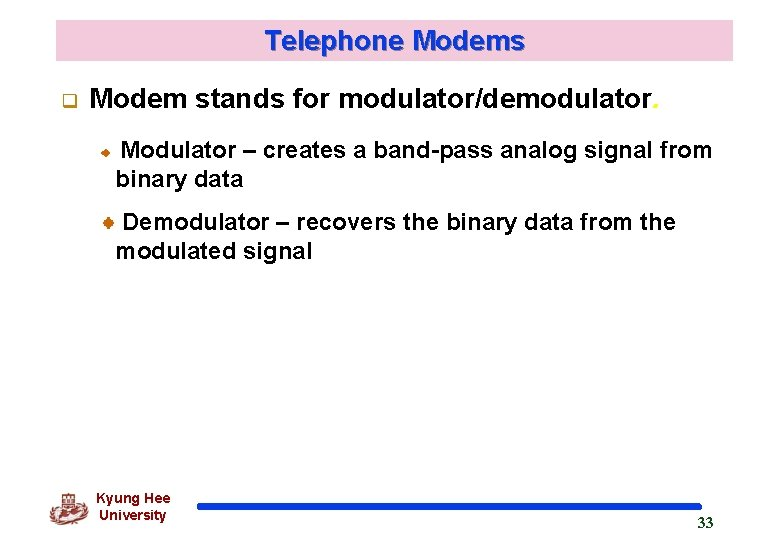Telephone Modems q Modem stands for modulator/demodulator. Modulator – creates a band-pass analog signal from binary data Demodulator – recovers the binary data from the modulated signal Kyung Hee University 33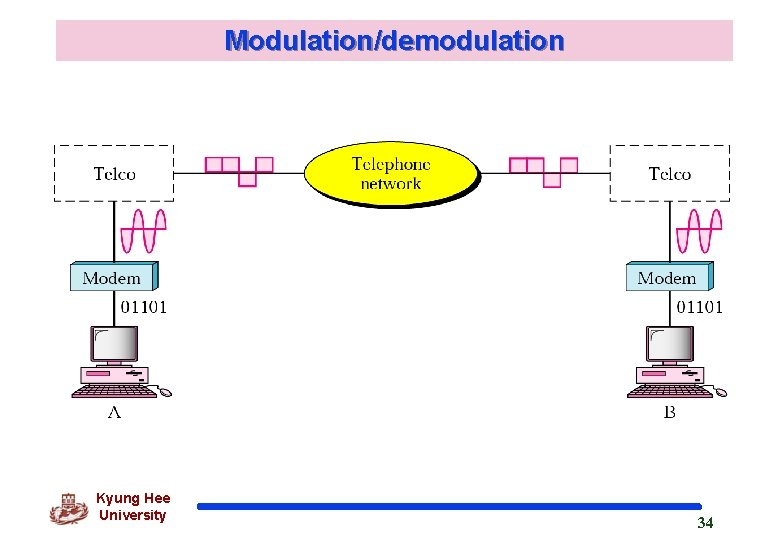Modulation/demodulation Kyung Hee University 34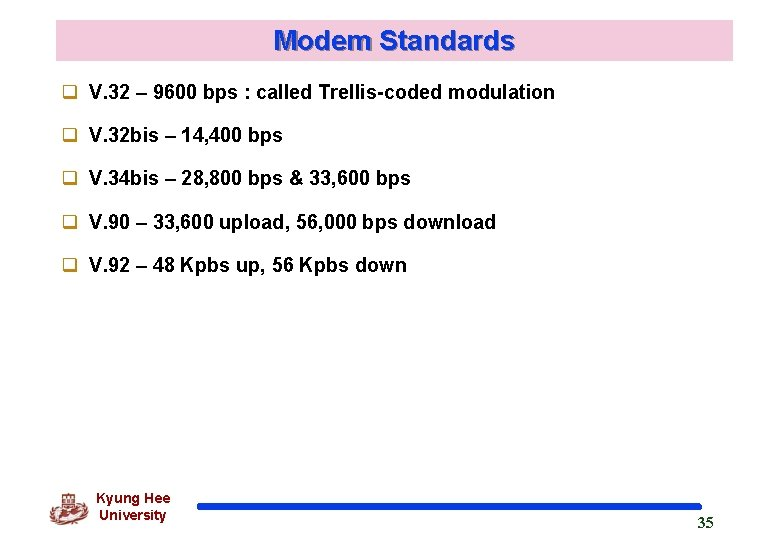Modem Standards q V. 32 – 9600 bps : called Trellis-coded modulation q V. 32 bis – 14, 400 bps q V. 34 bis – 28, 800 bps & 33, 600 bps q V. 90 – 33, 600 upload, 56, 000 bps download q V. 92 – 48 Kpbs up, 56 Kpbs down Kyung Hee University 35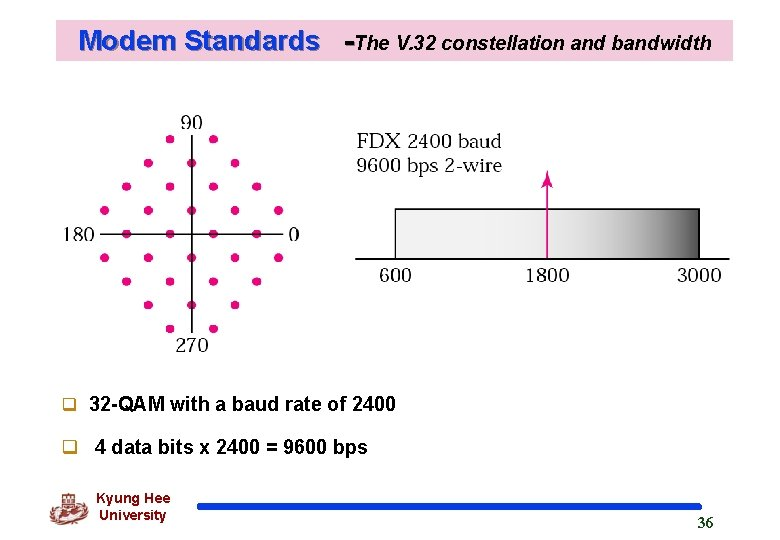Modem Standards -The V. 32 constellation and bandwidth q 32 -QAM with a baud rate of 2400 q 4 data bits x 2400 = 9600 bps Kyung Hee University 36Modem Standards -The V. 32 bis constellation and bandwidth • 128 -QAM • 6 data bits x 2400 baud = 14, 400 bps • fall-back, fall-forward feature enabling modem to adjust speed depending on line and/or signal quality Kyung Hee University 37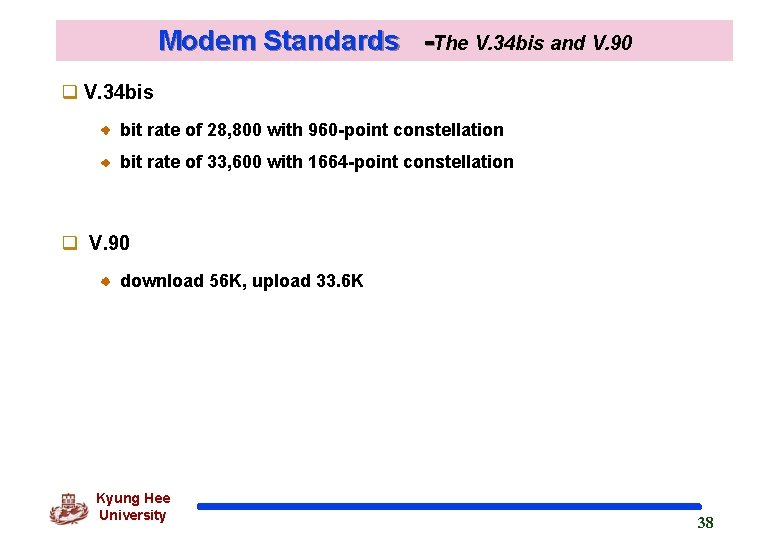Modem Standards -The V. 34 bis and V. 90 q V. 34 bis bit rate of 28, 800 with 960 -point constellation bit rate of 33, 600 with 1664 -point constellation q V. 90 download 56 K, upload 33. 6 K Kyung Hee University 38Traditional Modems q Kyung Hee University 39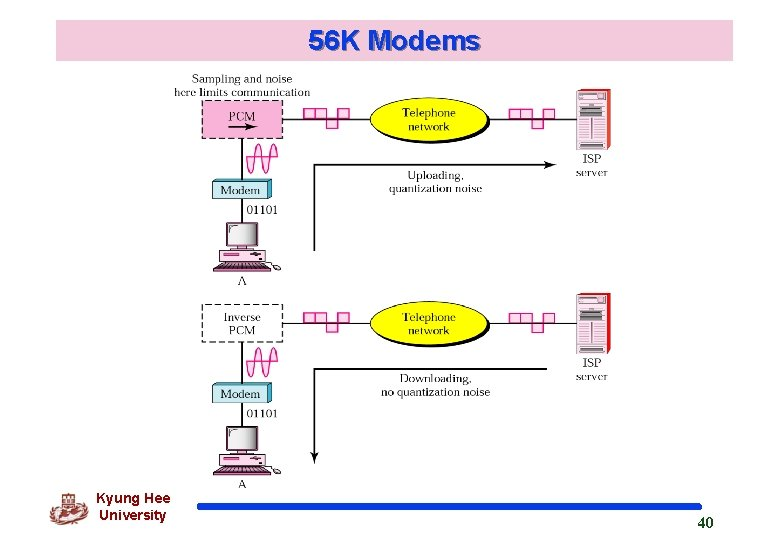56 K Modems Kyung Hee University 4056 K Modems q telephone company samples 8, 000 times per second q 8 bits per sample (7 data bits) q rate = 8, 000 x 7 = 56, 000 bps Kyung Hee University 41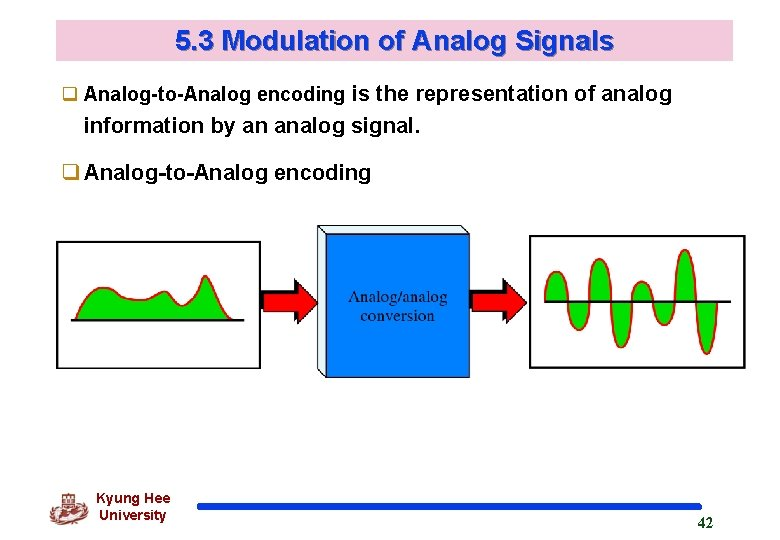5. 3 Modulation of Analog Signals q Analog-to-Analog encoding is the representation of analog information by an analog signal. q Analog-to-Analog encoding Kyung Hee University 42Analog-to-Analog 부호화(cont’d) q Type of analog-to-analog encoding Kyung Hee University 43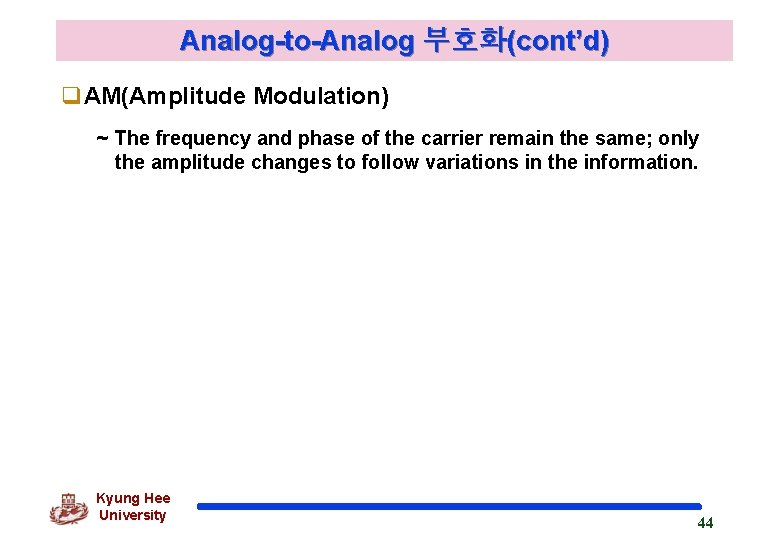Analog-to-Analog 부호화(cont’d) q. AM(Amplitude Modulation) ~ The frequency and phase of the carrier remain the same; only the amplitude changes to follow variations in the information. Kyung Hee University 44Analog-to-Analog 부호화(cont’d) q. Amplitude modulation Kyung Hee University 45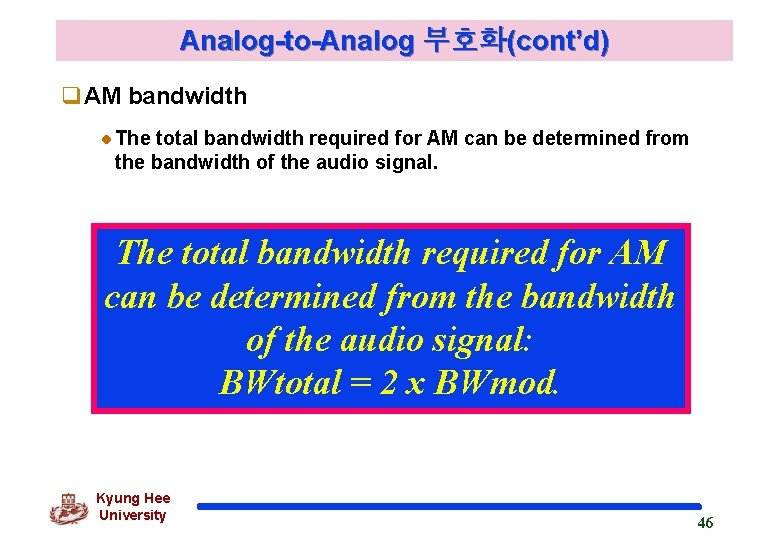Analog-to-Analog 부호화(cont’d) q. AM bandwidth The total bandwidth required for AM can be determined from the bandwidth of the audio signal: BWtotal = 2 x BWmod. Kyung Hee University 46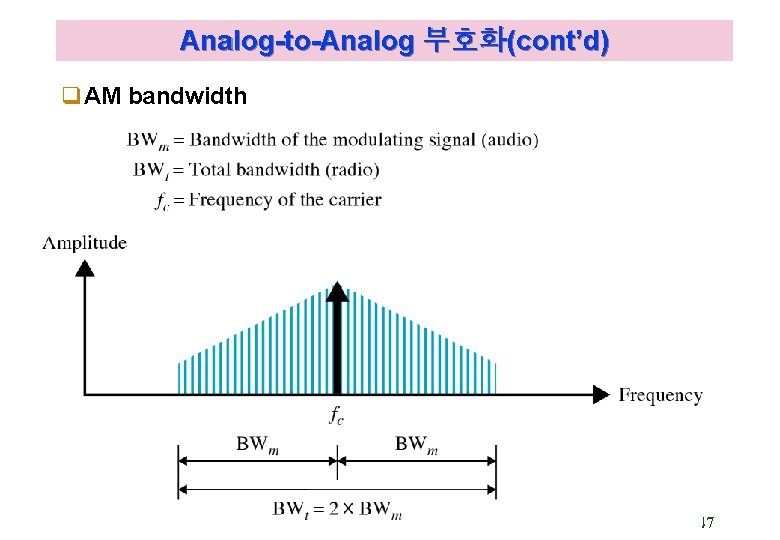Analog-to-Analog 부호화(cont’d) q. AM bandwidth Kyung Hee University 47Analog-to-Analog 부호화(cont’d) q Audio signal(음성과 음악) bandwidth : 5 KHz q Minimum bandwidth : 10 KHz (bandwidth for AM radio station) q AM stations are allowed carrier frequencies anywhere between 530 and 1700 KHz(1. 7 MHz) q each frequency must be separated by 10 KHz Kyung Hee University 48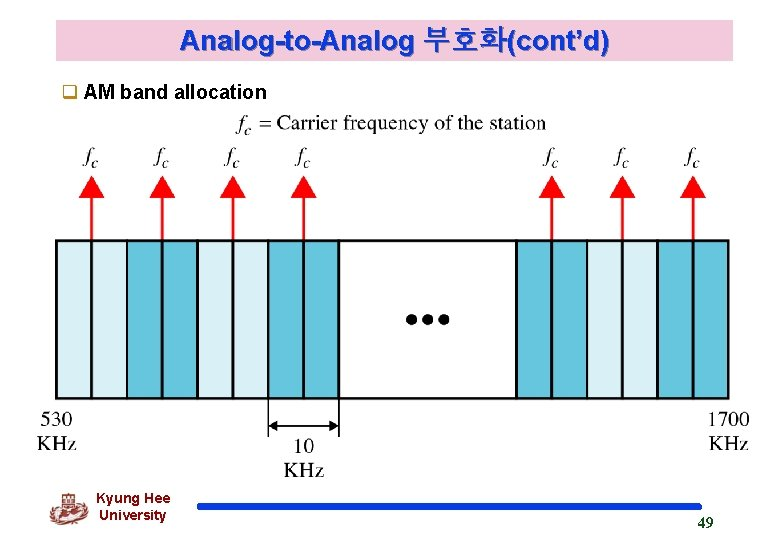Analog-to-Analog 부호화(cont’d) q AM band allocation Kyung Hee University 49Analog-to-Analog 부호화(cont’d) q We have an audio signal with a bandwidth of 4 KHz. What is the bandwidth needed if we modulate the signal using AM? Ignore FCC regulations. q An AM signal requires twice the bandwidth of the original signal: BW = 2 x 4 KHz = 8 KHz Kyung Hee University 50Analog-to-Analog 부호화(cont’d) q. FM(Frequency Modulation) as the amplitude of the information signal changes, the frequency of the carrier changes proportionately. Kyung Hee University 51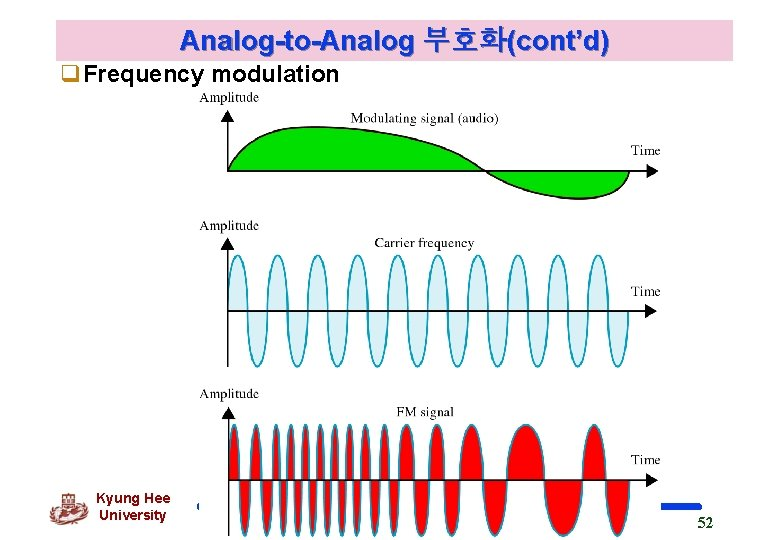Analog-to-Analog 부호화(cont’d) q. Frequency modulation Kyung Hee University 52Analog-to-Analog 부호화(cont’d) q. FM Bandwidth The bandwidth of an FM signal is equal to 10 times the bandwidth of the modulating signal. The total bandwidth required for FM can be determined from the bandwidth of the audio signal: BWt = 10 x BWm. Kyung Hee University 53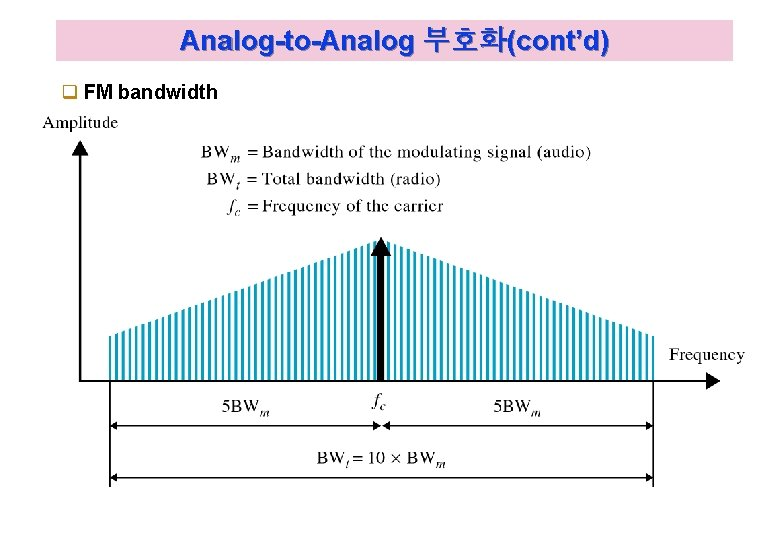Analog-to-Analog 부호화(cont’d) q FM bandwidth Kyung Hee University 54Analog-to-Analog 부호화(cont’d) q Bandwidth of an audio signal(음성과 음악) broadcast in stereo : 15 KHz q minimum bandwidth : 150 KHz q allows generally 200 KHz(0. 2 MHz) for each station q FM station are allowed carrier frequencies anywhere 88 and 108 MHz(each 200 KHz) Kyung Hee University 55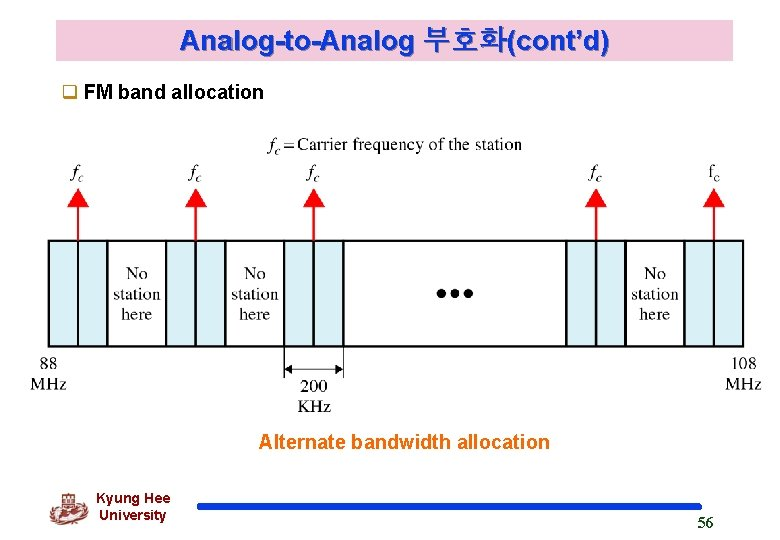Analog-to-Analog 부호화(cont’d) q FM band allocation Alternate bandwidth allocation Kyung Hee University 56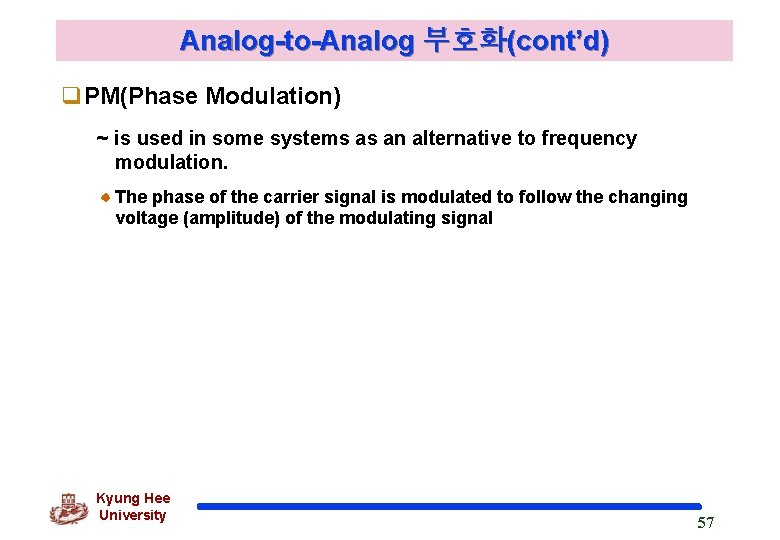Analog-to-Analog 부호화(cont’d) q. PM(Phase Modulation) ~ is used in some systems as an alternative to frequency modulation. The phase of the carrier signal is modulated to follow the changing voltage (amplitude) of the modulating signal Kyung Hee University 57